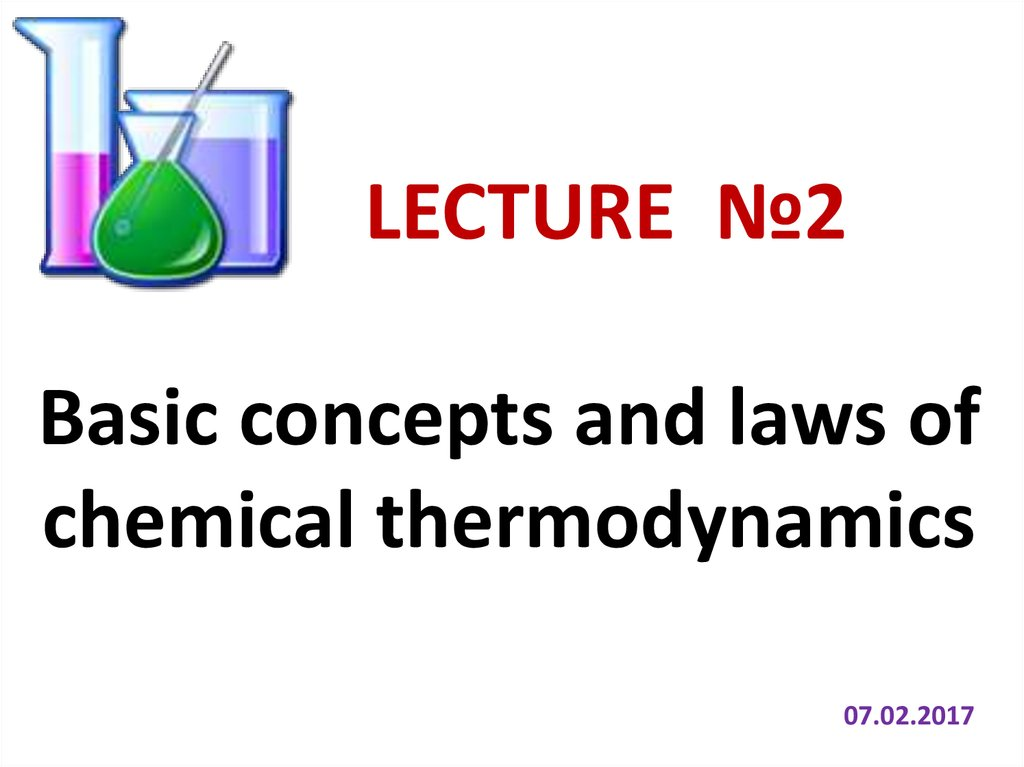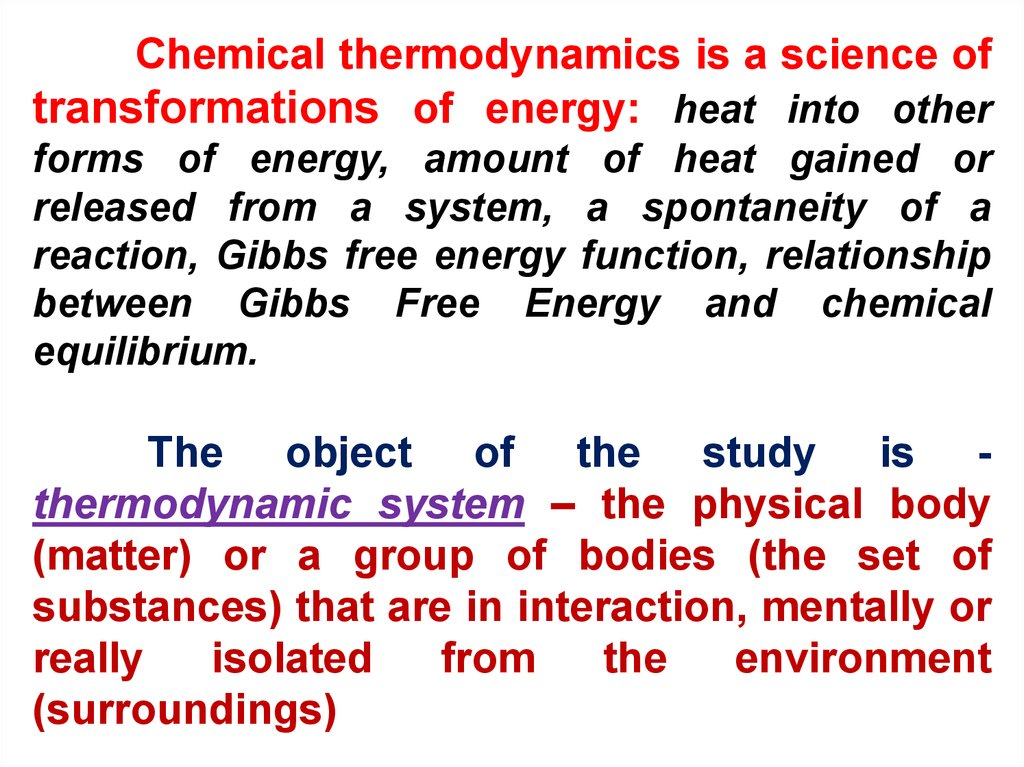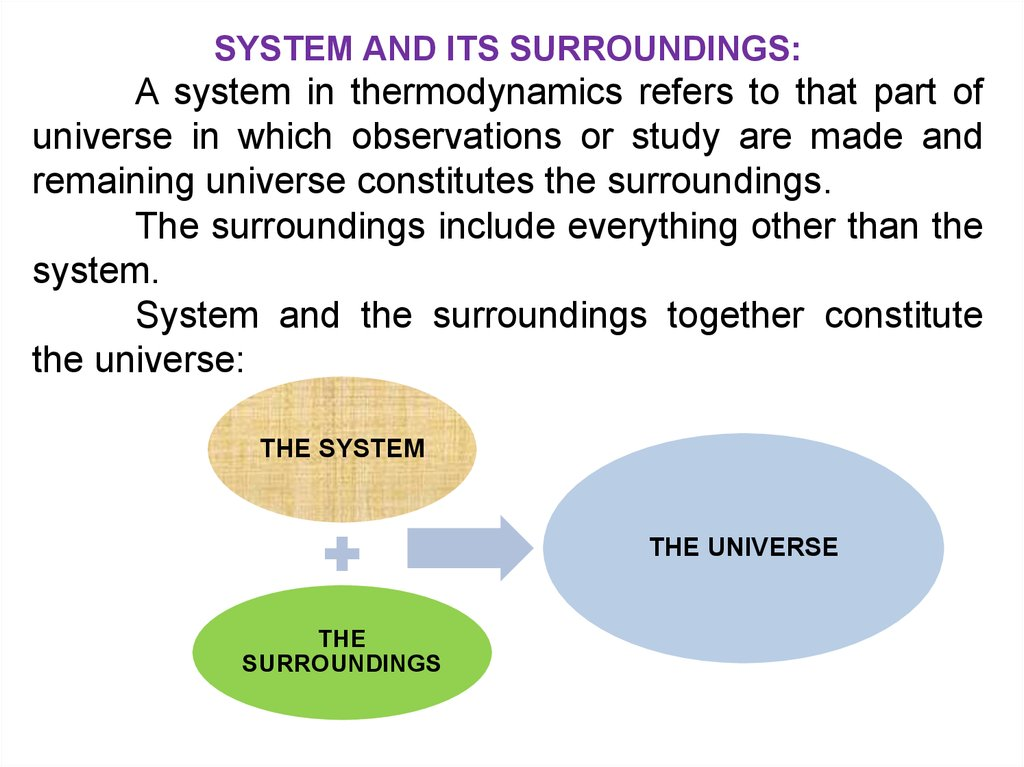# Basic concepts and laws of chemical thermodynamics

## 1.

LECTURE №2
Basic concepts and laws of
chemical thermodynamics
07.02.2017

## 2.

Chemical thermodynamics is a science of
transformations of energy: heat into other
forms of energy, amount of heat gained or
released from a system, a spontaneity of a
reaction, Gibbs free energy function, relationship
between Gibbs Free Energy and chemical
equilibrium.
The object of the study is thermodynamic system – the physical body
(matter) or a group of bodies (the set of
substances) that are in interaction, mentally or
really
isolated
from
the
environment
(surroundings)

## 3.

SYSTEM AND ITS SURROUNDINGS:
A system in thermodynamics refers to that part of
universe in which observations or study are made and
remaining universe constitutes the surroundings.
The surroundings include everything other than the
system.
System and the surroundings together constitute
the universe:
THE SYSTEM
THE UNIVERSE
THE
SURROUNDINGS

## 4.

surroundings
(environment)
SUBSTANCE
boundary
SYSTEM
boundary (surface)
ENERGY

## 5.

PHYSICAL STATES OF THE SYSTEM
HOMOGENEOUS
SYSTEM
Is a system without
surfaces separating the
different properties of
the system (phase)
HETEROGENEOUS
SYSTEM
Is a system, within which
there
is
a
surface
separating the different
properties of the system
PHASE is a set of homogeneous parts of a
heterogeneous system, with identical physical and
chemical properties, and separated from other parts
through visible surfaces

## 6.

water in an open beaker
is an open system as it
can
exchange
both
energy and matter with
the surrounding
Hot water in contact with
its vapour in a closed
container
Water in contact with its
vapour in a closed
insulated vessel is an
isolated system

## 7.

By the nature of the interaction with the
surroundings thermodynamic systems are
divided into 3 types:
• Open: Mass and Energy can transfer
between the System and the Surroundings
• Closed: Energy can transfer between the
System and the Surroundings, but not Mass
• Isolated: Neither Mass nor Energy can
transfer between the System and the
Surroundings

## 8.

The set of all physical and chemical properties
of the system describes its thermodynamic state.
State of the system is described by
thermodynamic parameters and functions.
All
quantities
characterizing
any
macroscopic property of the system is called
state parameters.
STATE
PARAMETERS
The extensive property
is proportional to the
system size and the
amount of material in the
system: m, V, n, E, S,G
An intensive property
does not depend on
the system size or the
amount of material in
the system: C, P, T,

## 9.

INTENSIVE PROPERTIES
EXTENSIVE PROPERTY
Energy
Entropy
Gibbs energy
Length
Mass
particle number
number of moles
Volume
electrical charge
Weight
• Chemical potential
• Concentration
• Density (or specific
gravity)
• Ductility
• Elasticity
• Hardness
• Melting point and
boiling point
• Pressure
• Specific energy
• Specific heat capacity
• Specific volume
• Spectral absorption
maxima (in solution)
• Temperature
• Viscosity

## 10.

Any change of parameter in the system
called the thermodynamic process.
Major Types of Thermodynamic Processes:
• Adiabatic process - a process with no heat
transfer into or out of the system.
• Isochoric process - a process with no change
in volume, in which case the system does no
work.
• Isobaric process - a process with no change
in pressure.
• Isothermal process - a process with no
change in temperature.

## 11.

THERMODYNAMIC PROCESS
ISOTHERMAL
(T=const)
ISOBARIC
(Р=const)
ISOCHORIC
(V=const)
(Q = 0)
No heat exchange between
the system and
the surroundings
Processes in which the system
returns to its original state
after a series of successive
transformations, called
cyclic process
or thermodynamic cycle

## 12.

Define Reversible and Irreversible Process
There are two main types of thermodynamic
processes: reversible process and the irreversible
process.
• Processes in which both the system and its
surroundings can be simultaneously returned to
their initial states after the process has been
completed are called a reversible process.
• Processes in which the system and its
surroundings cannot be simultaneously returned to
their initial states after the process has been
completed are called a irreversible.

## 13.

The main
processes in chemical
thermodynamics are:
•isobaric-isothermal (P, T = const)
•isochoric-isothermal (V, T = const)
All chemical reactions take place under
these conditions.

## 14.

Main objective of thermodynamics is to be able
to determine if a reaction will occur when reactants
are brought together under certain conditions.
Non-spontaneous Reaction – a reaction
does not occur under specific conditions.
Spontaneous Reaction – a reaction does
occur under specific conditions:
• A waterfall runs downhill
• A lump of sugar dissolves in a cup of coffee
• At 1 atm, water freezes below 0ºC and ice melts
above 0ºC
• Heat flows from a hotter object to a colder object
• Iron exposed to oxygen and water forms rust

## 15.

Derivative quantities, dependent on the
parameters of the system state and
immeasurable by direct methods are called
thermodynamic functions:
THERMODYNAMIC FUNCTIONS
PROCESS FUNCTIONS:
Q – HEAT,
A – WORK
STATE FUNCTIONS:
U – Internal energy,
H – Enthalpy,
S – Entropy,
G – Gibbs free energy,

## 16.

Function of the state is the total energy of the
system: E = K + P + U
In thermodynamics, state function is
a property of a system that depends only on the
current state of the system, not on the way in
which the system acquired that state. A state
function describes the equilibrium state of a system.
In contrast, process quantities – mechanical
work and heat are not properties of the system,
they characterize the process of energy exchange
between the system and the surroundings,
therefore
they
depend
on
the
path
(specific transition) of the process.

## 17.

In thermodynamics, the internal energy
(U) of a thermodynamic system is the total
kinetic energy due to the motion of
molecules
(translational,
rotational,
vibrational) and the potential energy
associated with the vibrational and electric
energy of atoms within molecules and
crystals. It includes the energy in all the
chemical bonds, and the energy of the free,
conduction electrons in metals.
U is a state function of a system, and is
a extensive quantity. The SI unit [U]=Joule.

## 18.

THE 1st LAW OF THERMODYNAMICS
The U is essentially defined by the 1st
law of thermodynamics which states that energy is
conserved «The increase in internal energy of a
closed system is equal to the difference of the heat
supplied to the system and the work done by it»:
U = Q – A
where:
• ΔU is the change in internal energy of a system
during a process;
• Q is heat "added to" a system (measured in joules
in SI);
• A is the mechanical work "done on" a system
(measured in joules in SI)

## 19.

Thus heat Q is given the system and consumed to
increase the internal energy U and to perform work
against external forces A:
Q = U + A
For isobar-isothermal process: p, Т = const
U = U2final — U1initial
Аgas = р V => V = V2final – V1 initial
Q = U + A
=>
Q = (U2 — U1) + (рV2 — рV1)
Q = (U2 + рV2 ) — (U1 + рV1)
=>
Q = - Н
U + pV = H – Enthalpy function

## 20.

The molar heat of formation of a
compound (ΔHf) is equal to its enthalpy change
(ΔH) when one mole of compound is formed at
25°C and 1 atm from elements in their stable
form.
Compound
H2O(l)
H2O(g)
H2O(s)
ΔHf (kJ/mol)
-285.8
-241.8
-291.85

## 21.

Experiment on determining heat (Q) of reaction for
MgSO4 (s) + 7H2O → MgSO4.7H2O

## 22.

A calorimeter is a
device
used
to
measure
the
quantity of heat flow
in
a
chemical
reaction. Two of the
most common types
of calorimeters are
the coffee cup
calorimeter
and
the
bomb
calorimeter.

## 23.

HESS’S LAW
• overall heat change of a chemical reaction is
independent of its pathway,
• energy change in changing A + B → C + D is the
same regardless of the route (independent of
route) by which the chemical changes occurs.
H
H
H
reac tan ts
chem reaction
products
aA bB cC dD
H H H H
B
D
A
C
H
( H d H c) ( H b H a)
D
B
C
A
chem rxn
Hess’s Law – used to calculate ΔH for reaction which cannot be
determined experimentally

## 25.

Q=– Н
If the reaction is generated heat (Q>0), enthalpy of
the system is lowered ( H<0), in this case the
reaction is called exothermic.
If the reaction is absorbed heat (Q<0), the system
increases the enthalpy ( H>0), and it called the
endothermic reaction.

Enthalpy of
reaction
using
combustion
data

## 27.

THE 2nd LAW OF THERMODYNAMICS
All
spontaneous
processes
are
irreversible (e.g. heat flows from hot to cold
spontaneously and irreversibly)
To predict spontaneity we need:
• Change in enthalpy.
• Entropy – a measure of the randomness or
disorder of a system: ↑ disorder = ↑ entropy

## 28.

1.
2.
3.
4.
Entropy
Entropy is a thermodynamic property that measures
the degree of randomization or disorder at
the microscopic level. The natural state of affairs is
for entropy to be produced by all processes.
A macroscopic feature which is associated with
entropy production is a loss of ability to do useful work.
Energy is degraded to a less useful form, and it is
sometimes said that there is a decrease in
the availability of energy.
Entropy is an extensive thermodynamic property. In
other words, the entropy of a complex system is the
sum of the entropies of its parts.
The notion that entropy can be produced, but never
destroyed, is the second law of thermodynamics.

## 29. Standard Entropy

The standard entropy of
reaction ( S0) is the
entropy change for a
reaction carried out at 1
atm and 250C:
S
S
S
reac tan ts
chem reaction
products

## 30. The Second Law of Thermodynamics

• The entropy of the universe increases in a
spontaneous process and remains unchanged in an
equilibrium process. (Clausius)
• "It is impossible in any way to diminish the entropy
of a system of bodies without there by leaving
behind changes in other bodies" (Planck)
• "In any irreversible process the total entropy of all
bodies concerned is increased." (Lewis)
Spontaneous process:
Suniv = Ssys + Ssurr > 0
Equilibrium process:
Suniv = Ssys + Ssurr = 0

## 31. The 3rd Law of Thermodynamics and Absolute Entropy

• The entropy of a perfect crystalline
substance is zero at the absolute
zero of temperature
Absolute zero is −273.15 °C (degrees
Celsius), or −459.67 °F (degrees
Fahrenheit) or 0 K (kelvin).

## 32. G – Gibbs Free Energy

• Predicts
the
direction
of
a
spontaneous reaction.
• Uses properties of the system to
calculate.
• For a constant pressure-temperature
process: GT = Hsys – T Ssys
G < 0
The reaction is spontaneous in the forward
direction.
G > 0
The reaction is nonspontaneous as written.
The reaction is spontaneous in the reverse direction.
G = 0
The reaction is at equilibrium.

## 33. Standard Free-Energy Changes

The standard free-energy of reaction ( G reac) is
the free-energy change for a reaction when it occurs
under standard-state conditions.
Standard free energy of formation ( G ) is the
free-energy change that occurs when 1 mole of the
compound is formed from its elements in their
standard states.
G
G
G
reac tan ts
chem reaction
products

## 35.

Thermodynamic Equilibrium
A system is said to be at thermodynamic
equilibrium when all of its macroscopic properties
are time-independent and remain so when the
system is isolated from its surroundings.
Thermodynamic equilibrium, in simple words is,
same temperature. At same temperature bodies do
exchange heat but do not gain or lose heat.
The system is said to be in thermodynamic
equilibrium if the conditions for following three equilibrium
is satisfied:
1) Mechanical equilibrium
2) Chemical equilibrium
3) Thermal equilibrium

## 36.

0eth Law of Thermodynamics:
Two systems in thermal equilibrium
with a third system are in thermal
equilibrium to each other.

## 37. Free Energy and Chemical Equilibrium

G = G0 + RT lnq
R is the gas constant (8.314 J/K•mol)
T is the absolute temperature (K)
q is the reaction quotient
K is the equilibrium constant of reaction
At Equilibrium: G = 0 => q = K
0 = G0 + RT lnK
G0 = – RT lnK

## 39. QUIZ ME

1
A pressure cooker reduces cooking time
for food because …
heat is more evenly distributed in the cooking
space
boiling point of water involved in cooking is
increased
the higher pressure inside the cooker crushed the
food materia
cooking involves chemical changes helped by a
rise in temperature
NEXT

## 40. QUIZ ME

2
Which one is not a state function?
Internal energy (E)
Enthalpy
Heat (q)
Volume
NEXT

## 41. QUIZ ME

3
When no heat energy is allowed to
enter or leave the system, it is called:
Irreversible process
Reversible process
Isothermal process
NEXT

## 42. QUIZ ME

4
contains some ice cubes. This is an
example of a …
Isolated system
Closed system
Open system
Non-thermodynamics system

## 43.

GLOSSARY OF LECTURE
1. Thermodynamics: Energy differences and transfers between systems.
2. Systems:
• Isolated system: “have walls or boundaries that are rigid, do not permit
transfer of mechanical energy, perfectly insulating, and impermeable. The have
a constant energy and mass content.
• Adiabatic systems: Perfectly insulated systems.
• Closed systems: have walls that allow transfer of energy in or out of the
system but are impervious to matter. They contain a fixed mass and
composition, but variable energy.
• Open Systems: have walls that allow transfer of both energy and matter to
and from the system.
3. Equilibrium: “A system at equilibrium has none of its properties changing
with time”. A system at equilibrium will return to that state after being disturbed.
4. State Variables: Variables that define the state of a system.
• Extensive variables are proportional to the quantity of matter being
considered (V, total Cp).
• Intensive variables are independent of quantity (concentration, viscosity,
density, molar Cp)

## 44.

5. Work (w): “The transfer of energy from one mechanical system to
another. It is always completely convertible to the lifting of a weight”. “The
energy that flows across a system boundary in response to a force moving
through a distance (such as happens when a system changes volume”.
6. Heat (q):
• “The transfer of energy that results from temperature differences”.
• “The energy that flow across a system boundary in response to a
• “That part of any energy transfer that is not accounted for by mechanical
work (FxD).”
• q=∆U-w
7. Heat Capacity: The relation between heat transferred to a body and the
change in T.
8. Enthalpy: The increase in enthalpy of a system is equal to the heat
absorbed at constant pressure, assuming the system does only PV work.
9. Entropy: A measure of the loss of capacity of the system to do work.
10. Gibbs free energy is a measure of the potential for reversible or maximum
work that may be done by a system at constant temperature and pressure. It is a
thermodynamic property that was defined in 1876 by Josiah Willard Gibbs to
predict whether a process will occur spontaneously at constant temperature and
pressure.

## 45.

11. ZEROTH LAW: “Two bodies in thermal equilibrium with a third are in
thermal equilibrium with each other” (Basis of the concept of
temperature)
12. FIRST LAW: “The alegraic sum of all energy changes in an isolated
system is zero” (Conservation of energy). Energy can be converted from
one form to another but cannot be created or destroyed. ∆U=q+w. This
is based only on observation
13. SECOND LAW: “Spontaneous changes are those which, if carried
out under the proper conditions, can be made to do work. If carried out
reversibly they yield a maximum amount of work. In natural processes,
maximum work is never obtained.
14. THIRD LAW:
• First expressed as Nernst's Heat Theorem: “Nernst (1905): As T → 0
K , ΔS → 0 for all isothermal processes in condensed phases”
• More general and useful formulation by M. Planck: “Planck (1911): As
T → 0 K , S → 0 for every chemically homogeneous substance in a
perfect crystalline state ”
• This leads to the following interesting corollary: “It is impossible to
decrease the temperature of any system to T = 0 K in a finite number of
steps ”